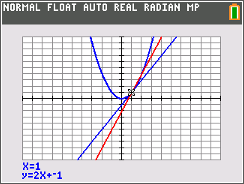### Secant Lines

In this activity, students will observe the slopes of the secant and tangent line as a point on the function approaches the point of tangency.
•TI-84 Plus CE
•TI-84 Plus C Silver Edition
• TI-84 Plus Silver Edition
• TI-84 Plus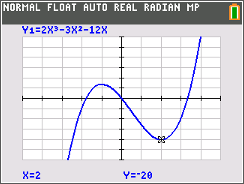### Extrema and Concavity

Students learn how to find and label extrema using first and second derivatives, be able to inspect a graph and determine which extrema the function has, and be able to use trace, fMin, and fMax to verify the computed answers and find critical values for parametric functions.
•TI-84 Plus CE
•TI-84 Plus C Silver Edition
• TI-84 Plus Silver Edition
• TI-84 Plus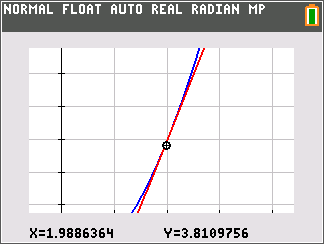### Zooming In On Local Linearity

In this activity, students will explore the behavior of various functions by zooming in on their graphs. They will investigate the concept of local linearity.
•TI-84 Plus CE
•TI-84 Plus C Silver Edition
• TI-84 Plus Silver Edition
• TI-84 Plus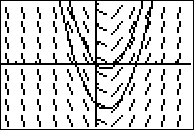### Using Slope Fields

Students identify whether a slope field approximately reflects a differential equation. They also determine whether a potential solution fits the slope field.
• TI-84 Plus Silver Edition
• TI-84 Plus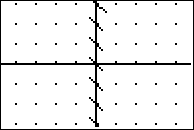### Introduction to Slope Fields

In this activity, students learn what a slope field represents in terms of dy/dx. Students create a slope field for a given differential equation
•TI-84 Plus CE
•TI-84 Plus C Silver Edition
• TI-84 Plus Silver Edition
• TI-84 Plus### Investigating the Derivatives of Some Common Functions

In this activity, students will investigate the derivatives of sine, cosine, natural log, and natural exponential functions by examining the symmetric difference quotient at many points. They develop the idea of the derivative as a function. They gather evidence toward some common derivative formulas. They also use numerical and graphical investigations to form conjectures.
•TI-84 Plus CE
•TI-84 Plus C Silver Edition
• TI-84 Plus Silver Edition
• TI-84 Plus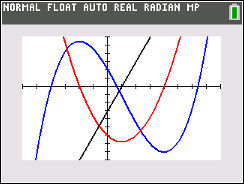### Graphing Relationships

In this activity, students explore information about a graph based on the first and second derivatives. They learn that a function's derivative is positive when the function increases and negative when the function decreases. They learn that the second derivative is positive when the graph is concave upward and negative when the graph is concave downward.
•TI-84 Plus CE
•TI-84 Plus C Silver Edition
• TI-84 Plus Silver Edition
• TI-84 Plus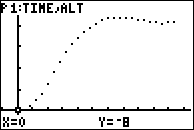••